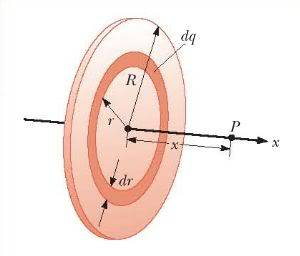# The Electric Field of a Uniformly Charged Disk

## Homework Statement

A disk of radius R has a uniform surface charge density σ. Calculate the electric field at a point P that lies along the central perpendicular axis of the disk and a distance x from the center of the disk.## Homework Equations

Electric field due to a continuous charge distribution:

ke$\int$$\frac{dq}{r^{2}}$$\hat{r}$

Surface charge density:

σ = $\frac{Q}{A}$

## The Attempt at a Solution

This is an example problem from my book, so I already know the solution. The real question I have is how they go about solving it. For the most part, it makes sense, but there is one small step in their calculation that I am confused by.

They setup their formula as thus: Ex = kex$\pi$σ$\int$$\frac{2rdr}{(r^{2}+x^{2})^{3/2}}$. This I understand, but their next step is what throws me off. They rewrite this as:

kex$\pi$σ$\int$(r$^{2}$+x$^{2}$)$^{-3/2}$d(r$^{2}$)

I assume that the d(r2) refers to an infinitesimal value of r2. Yet, what I'm confused by is if this is a normal operation in calculus (for I've never seen it before)? In other words, for any other integrations where you have, say, ∫xdx, could this be rewritten as ∫dx2? More generally, could we treat any integration by way of the power rule like so: ∫xmd(xn), with the solution being $\frac{x^{m+n}}{m+n}$ + C? Or am I completely misinterpreting the calculation?

Thanks!

SammyS
Staff Emeritus
Homework Helper
Gold Member

## Homework Statement

A disk of radius R has a uniform surface charge density σ. Calculate the electric field at a point P that lies along the central perpendicular axis of the disk and a distance x from the center of the disk.## Homework Equations

Electric field due to a continuous charge distribution:

ke$\int$$\frac{dq}{r^{2}}$$\hat{r}$

Surface charge density:

σ = $\frac{Q}{A}$

## The Attempt at a Solution

This is an example problem from my book, so I already know the solution. The real question I have is how they go about solving it. For the most part, it makes sense, but there is one small step in their calculation that I am confused by.

They setup their formula as thus: Ex = kex$\pi$σ$\int$$\frac{2rdr}{(r^{2}+x^{2})^{3/2}}$. This I understand, but their next step is what throws me off. They rewrite this as:

kex$\pi$σ$\int$(r$^{2}$+x$^{2}$)$^{-3/2}$d(r$^{2}$)

I assume that the d(r2) refers to an infinitesimal value of r2. Yet, what I'm confused by is if this is a normal operation in calculus (for I've never seen it before)? In other words, for any other integrations where you have, say, ∫xdx, could this be rewritten as ∫dx2? More generally, could we treat any integration by way of the power rule like so: ∫xmd(xn), with the solution being $\frac{x^{m+n}}{m+n}$ + C? Or am I completely misinterpreting the calculation?

Thanks!
$\displaystyle d\,\left(f(x)\right)=f'(x)\,dx$

So, $\displaystyle d(r^2)=\left(\frac{d}{dr}(r^2)\right)dr=2r\,dr\,.$